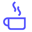a concise shot of dev knowledge
Become a Contributor
Concise shots of dev knowledge

RELATED TAGS

merge
sorting
java
divide
conquer

# How to implement a merge sort in JavaEdpresso Team

A merge sort is a type of a divide and conquer algorithm used to sort a given array; this means that the array is divided into halves and then further sub-divided till division can no longer take place.

This happens when you reach a single element array as that has no middle to further divide the array on. Each divided sub-array is then sorted and subsequently merged with other sub-divisions. The sorted merge continues till all divisions of the array have been merged, giving us a sorted array.



## How does a merge sort work?

Below is an illustration that shows the basic outline:

Given an array, we need to sort it using Merge Sort. Hence, we must divide the array into half
1 of 7

## Implementation

Now that we know how the algorithm works in theory, let’s see how to write the code to implement it.

class sorting {

public static void merge(int[] left_arr,int[] right_arr, int[] arr,int left_size, int right_size){

int i=0,l=0,r = 0;
//The while loops check the conditions for merging
while(l<left_size && r<right_size){

if(left_arr[l]<right_arr[r]){
arr[i++] = left_arr[l++];
}
else{
arr[i++] = right_arr[r++];
}
}
while(l<left_size){
arr[i++] = left_arr[l++];
}
while(r<right_size){
arr[i++] = right_arr[r++];
}
}

public static void mergeSort(int [] arr, int len){
if (len < 2){return;}

int mid = len / 2;
int [] left_arr = new int[mid];
int [] right_arr = new int[len-mid];

//Dividing array into two and copying into two separate arrays
int k = 0;
for(int i = 0;i<len;++i){
if(i<mid){
left_arr[i] = arr[i];
}
else{
right_arr[k] = arr[i];
k = k+1;
}
}
// Recursively calling the function to divide the subarrays further
mergeSort(left_arr,mid);
mergeSort(right_arr,len-mid);
// Calling the merge method on each subdivision
merge(left_arr,right_arr,arr,mid,len-mid);
}

public static void main( String args[] ) {
int [] array = {12,1,10,50,5,15,45};
mergeSort(array,array.length);
for(int i =0; i< array.length;++i){
System.out.print(array[i]+ " ");
}
}
}

## Understanding Implementation

The code above shows how to write Mergesort in Java. Now let’s understand the code in detail.

The implementation of Merge Sort given above is a recursive one. You start by calling the mergeSort method as shown on line 51 with the following input parameters:

• The array to be sorted
• The length of the array to be sorted

Now let’s look at the recursive method, mergeSort which is written from lines 24 to 47.

• The method first checks the base case which is, that if the length of the array is less than 2 then return the array as it is, as shown on line 25.

• If the base case is not satisfied then the method creates two arrays; the left and right subdivision and recursively calls itself on these two arrays which now contain the copied data of the original array

• The last step, on line 46 is that the method calls the merge method which compares and sorts the left and right arrays.

Now let’s discuss the merge method written from lines 3 to 22.

The function takes in many parameters; the left array, the right array, the actual array, the sizes of the left and right array.

Now let’s see how the method proceeds:

• The first while loop from lines 7 to 15 checks if the value of both the iterators is less than the respective array size, then it compares which of the element in right_arr or left_arr is smaller than the other and chooses that one to place in arr.

• The second while loop from lines 16 to 18 checks that if only elements in the left_arr are left then simply append them to arr

• The third while loop from lines 19 to 21 does the same but for the right_arr.

RELATED TAGS

merge
sorting
java
divide
conquer
RELATED COURSES

View all Courses

Keep Exploring

Learn in-demand tech skills in half the time